SLVSB71E February   2012  – September 2016

PRODUCTION DATA.

1. Features
2. Applications
3. Description
1.     Device Images
4. Revision History
5. Device Comparison Table
6. Pin Configuration and Functions
7. Specifications
8. Detailed Description
1. 8.1 Overview
2. 8.2 Functional Block Diagram
3. 8.3 Feature Description
4. 8.4 Device Functional Modes
9. Application and Implementation
1. 9.1 Application Information
2. 9.2 Typical Application
1. 9.2.1 Design Requirements
2. 9.2.2 Detailed Design Procedure
1. 9.2.2.1 Programming the Output Voltage
2. 9.2.2.2 External Component Selection
1. 9.2.2.2.1 Inductor Selection
2. 9.2.2.2.2 Capacitor Selection
3. 9.2.2.3 Tracking Function
4. 9.2.2.4 Output Filter and Loop Stability
3. 9.2.3 Application Curves
3. 9.3 System Examples
10. 10Power Supply Recommendations
11. 11Layout
12. 12Device and Documentation Support
13. 13Mechanical, Packaging, and Orderable Information

• RGT|16
• RGT|16

#### 9.2.3 Application Curves

VIN = 12 V, VOUT = 3.3 V, TA = 25°C, (unless otherwise noted)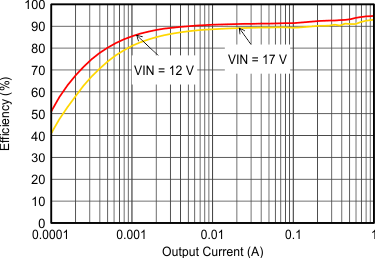Figure 8. Efficiency With 1.25 MHz, Vout = 5 V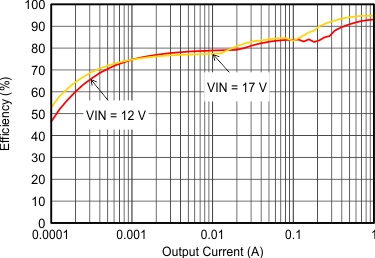Figure 10. Efficiency With 2.5 MHz, Vout = 5 V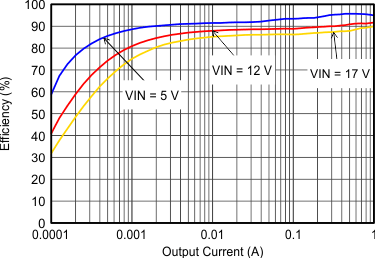Figure 12. Efficiency With 1.25 MHz, Vout = 3.3 V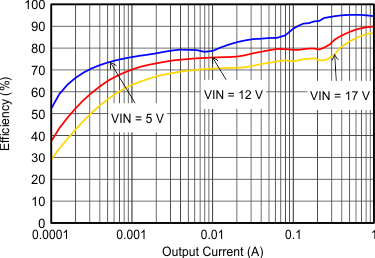Figure 14. Efficiency With 2.5 MHz, Vout = 3.3 V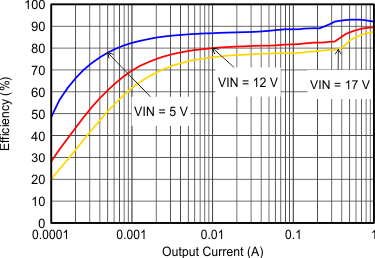Figure 16. Efficiency With 1.25 MHz, Vout = 1.8 V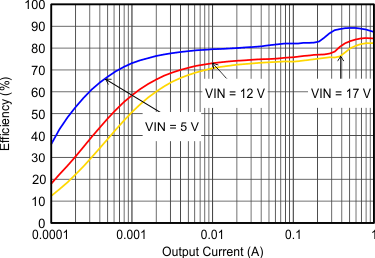Figure 18. Efficiency With 1.25 MHz, Vout = 0.9 V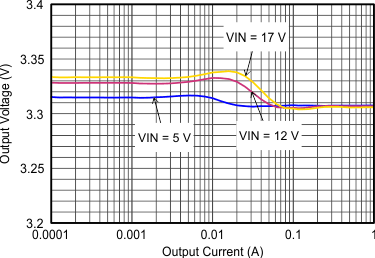Figure 20. Output Voltage Accuracy (Load Regulation)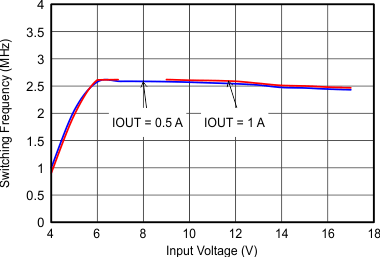FSW=Low
Figure 22. Switching Frequency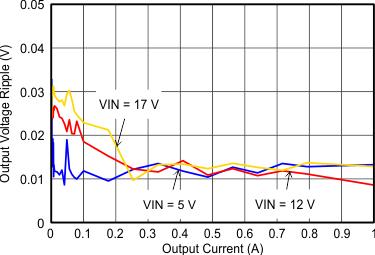Figure 24. Output Voltage Ripple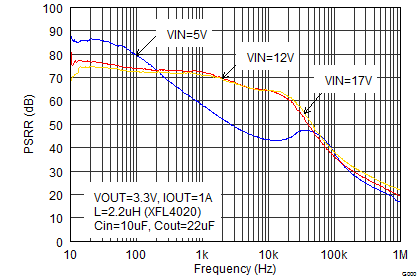IOUT = 1 A
Figure 26. Power Supply Rejection Ratio, FSW = 2.5 MHz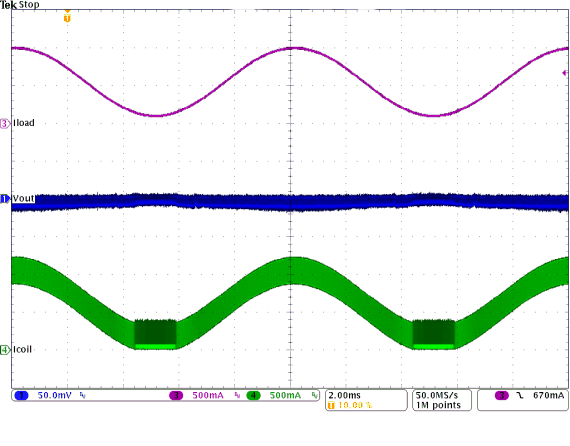Figure 28. PWM-PSM-Transition
(VIN = 12 V, VOUT = 3.3 V With 50 mV/div)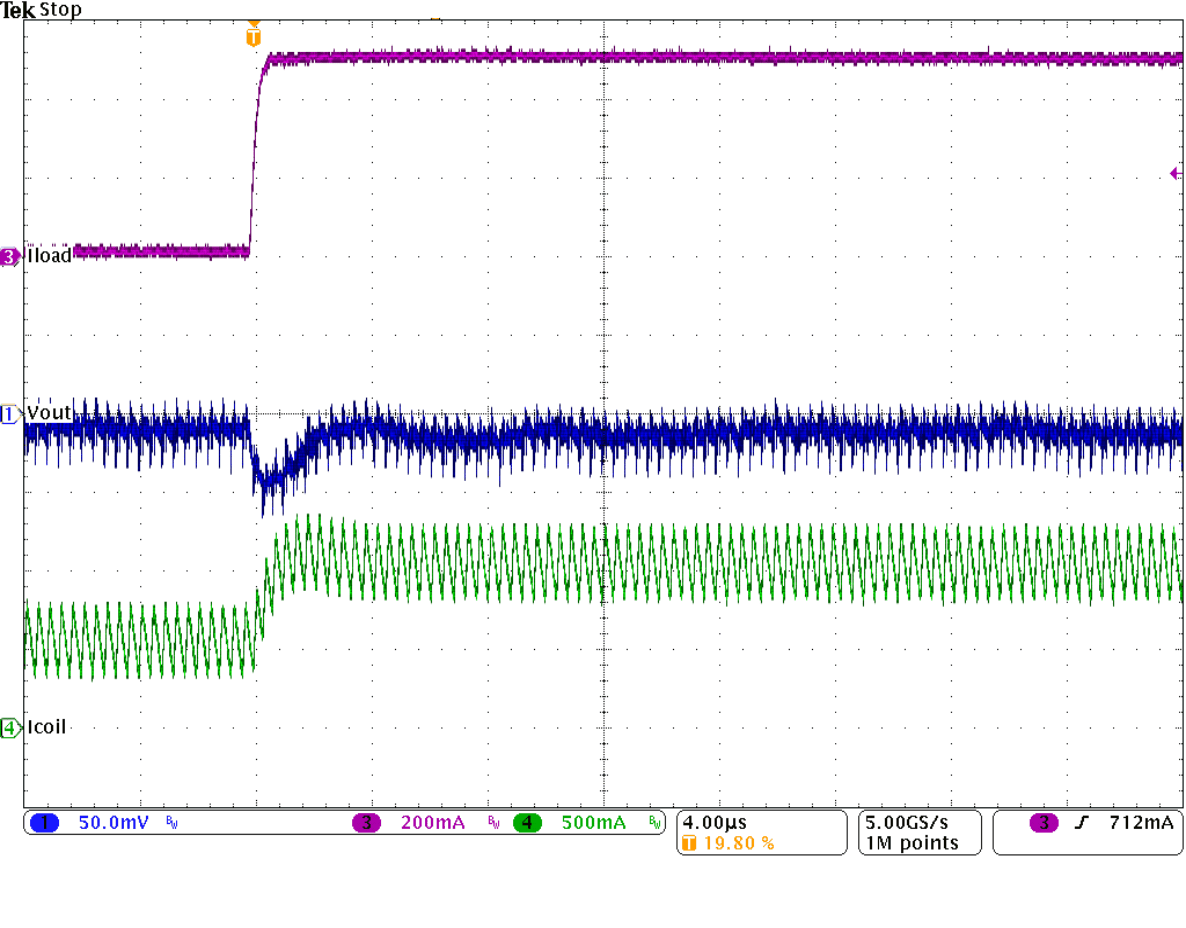Figure 30. Load Transient Response of Figure 29,
Rising Edge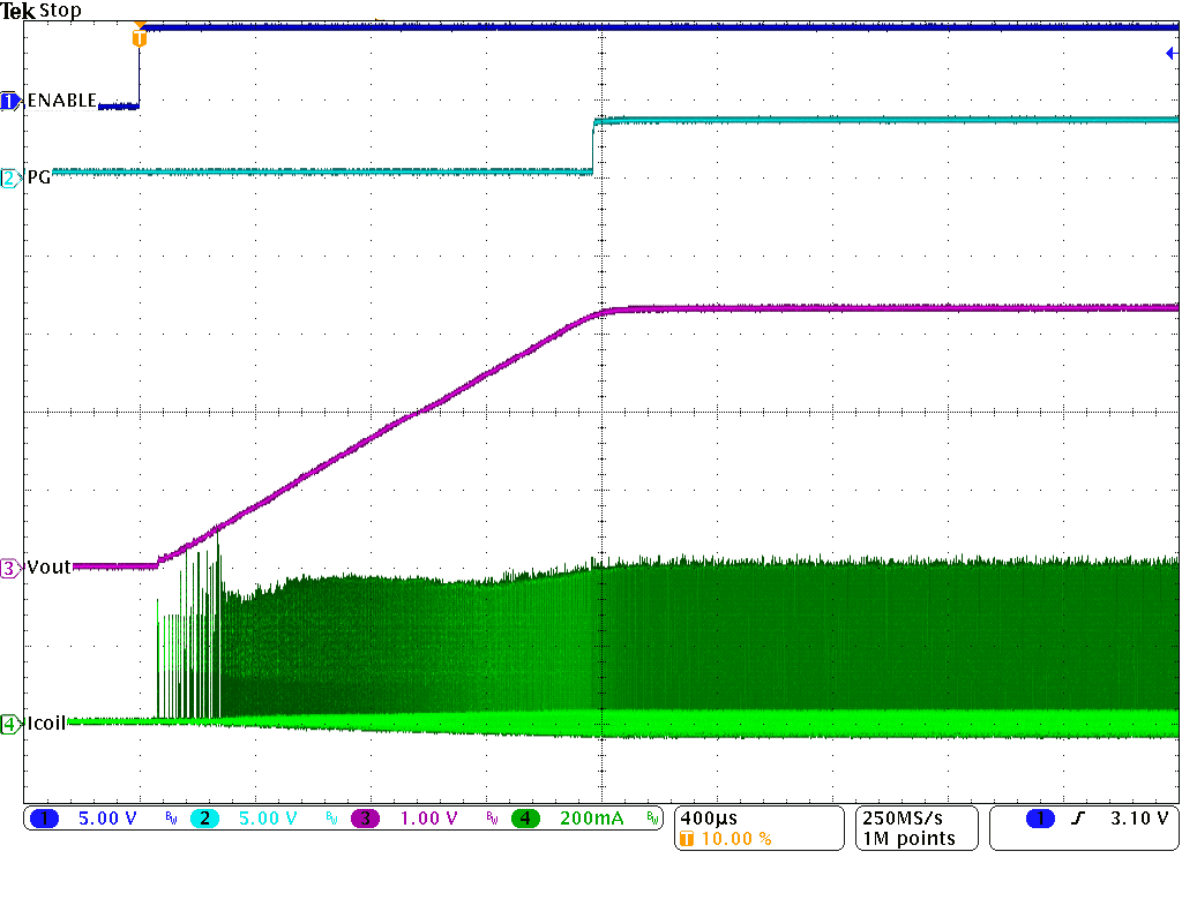Figure 32. Startup Into 100 mA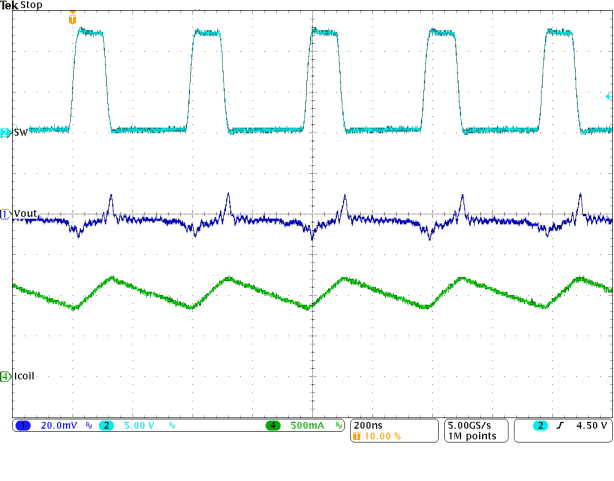Figure 34. Typical Operation In PWM Mode
(IOUT = 1 A)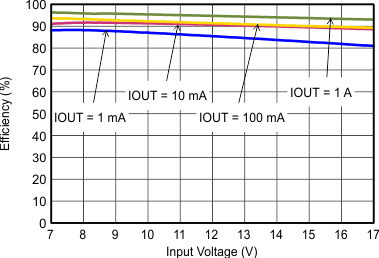Figure 9. Efficiency With 1.25 MHz, Vout = 5 V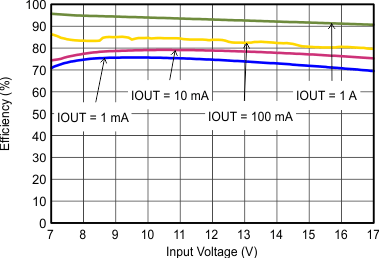Figure 11. Efficiency With 2.5 MHz, Vout = 5 V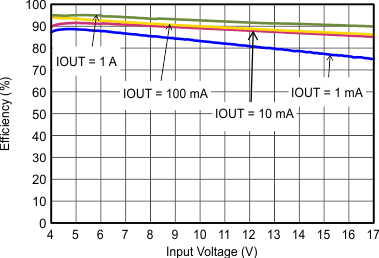Figure 13. Efficiency With 1.25 MHz, Vout = 3.3 V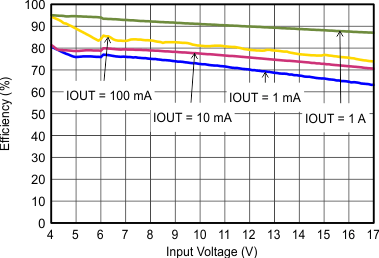Figure 15. Efficiency With 2.5 MHz, Vout = 3.3 V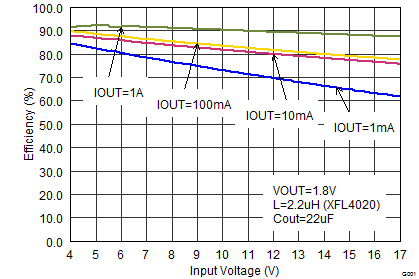Figure 17. Efficiency With 1.25 MHz, Vout = 1.8 V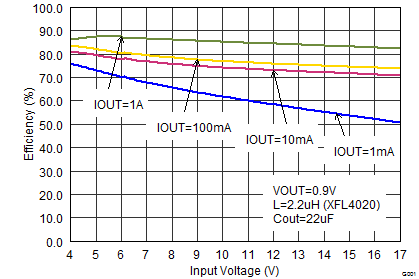Figure 19. Efficiency With 1.25 MHz, Vout=0.9 V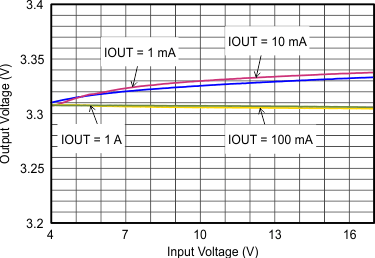Figure 21. Output Voltage Accuracy (Line Regulation)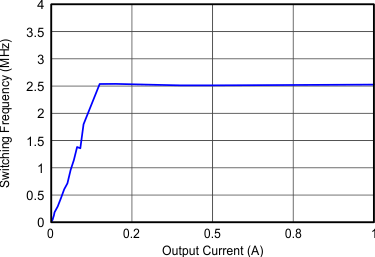FSW=Low
Figure 23. Switching Frequency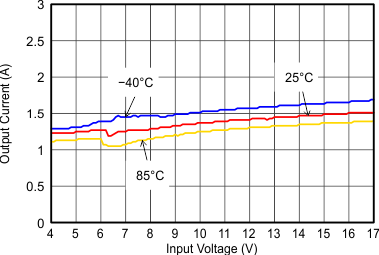Figure 25. Maximum Output Current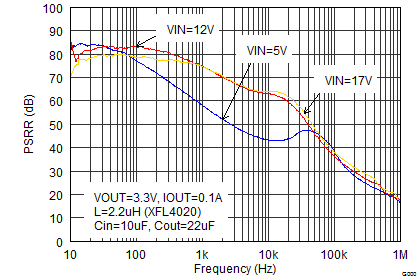IOUT = 0.1 A
Figure 27. Power Supply Rejection Ratio, FSW = 2.5 MHz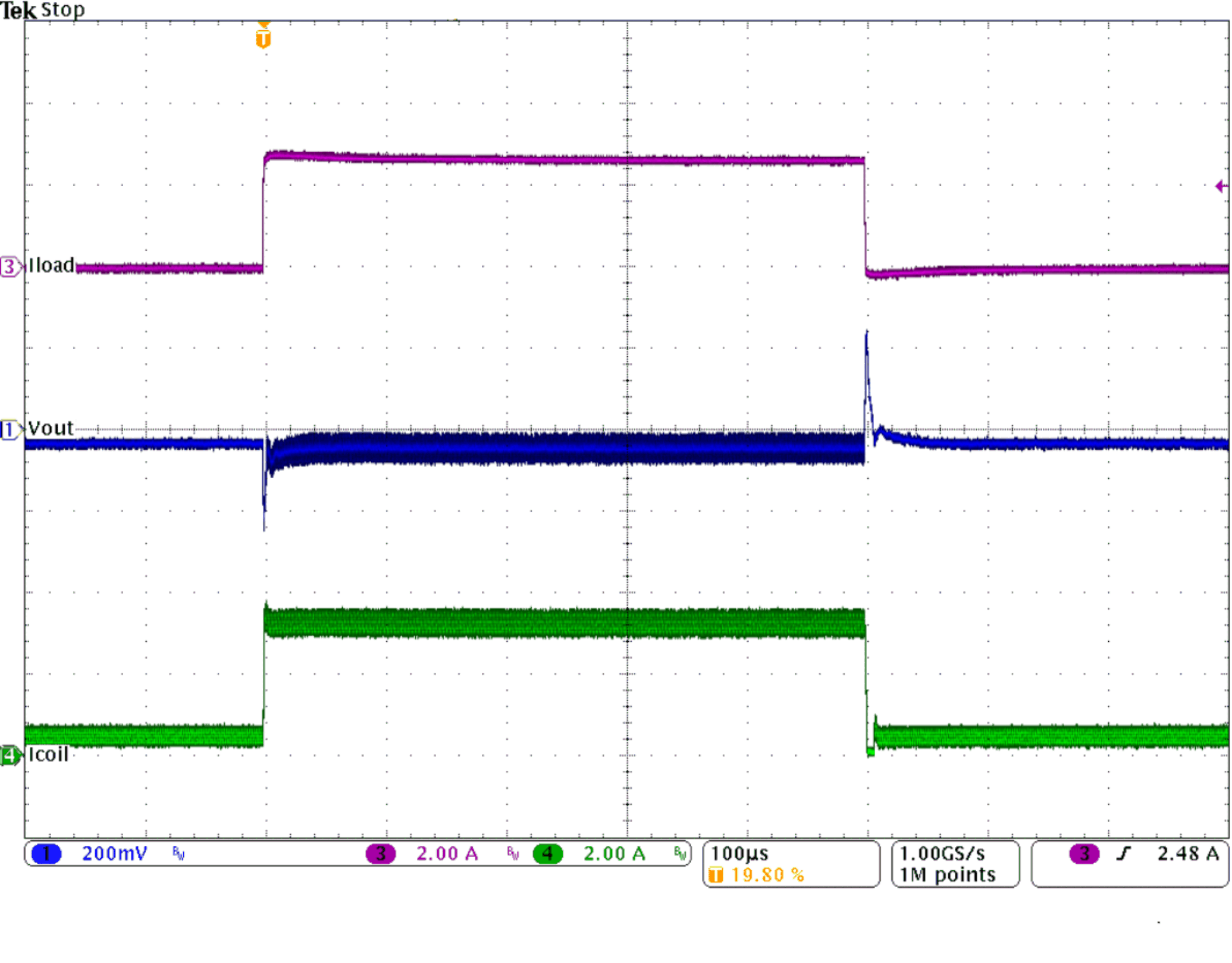Figure 29. Load Transient Response
(IOUT= 0.5 to 1 to 0.5 A, VIN = 12 V, VOUT = 3.3 V)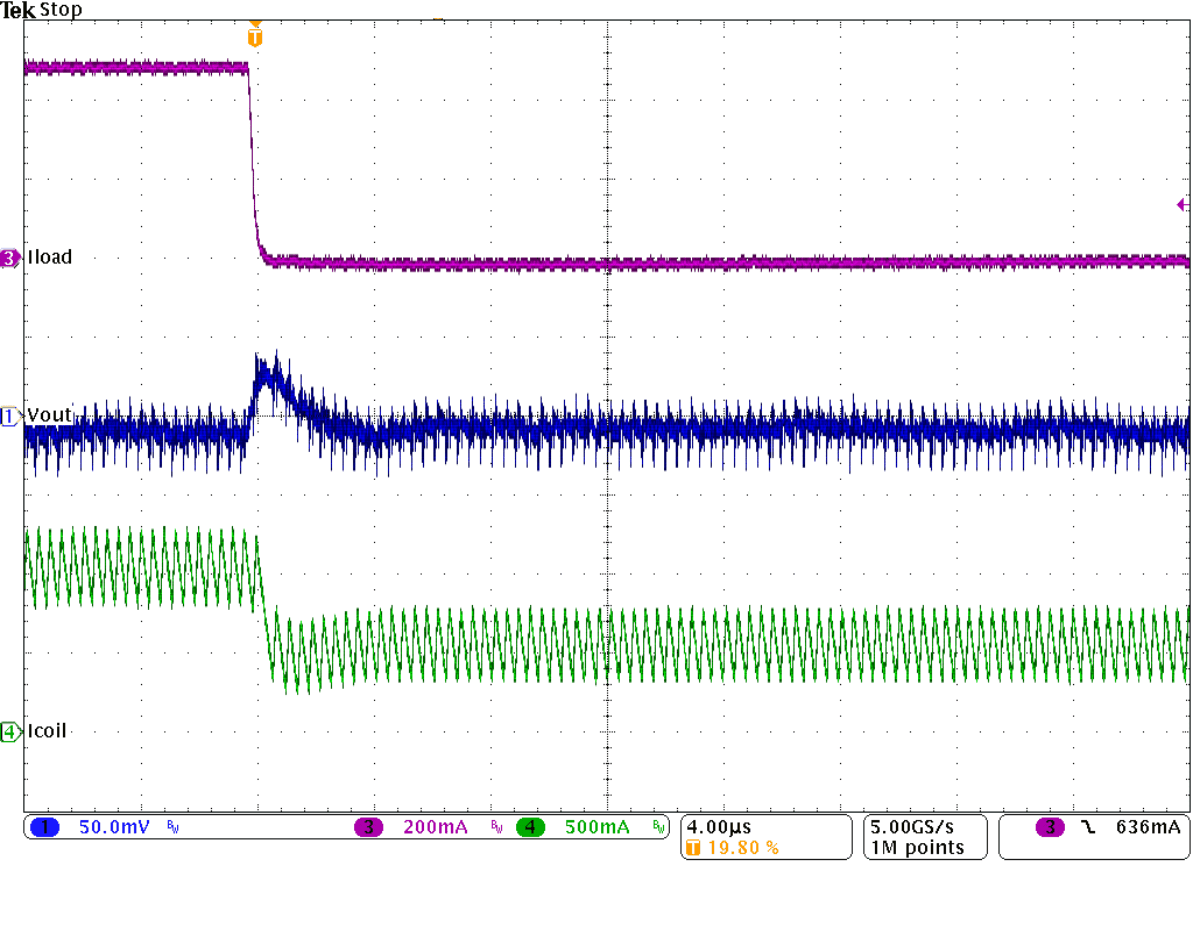Figure 31. Load Transient Response of Figure 29,
Falling Edge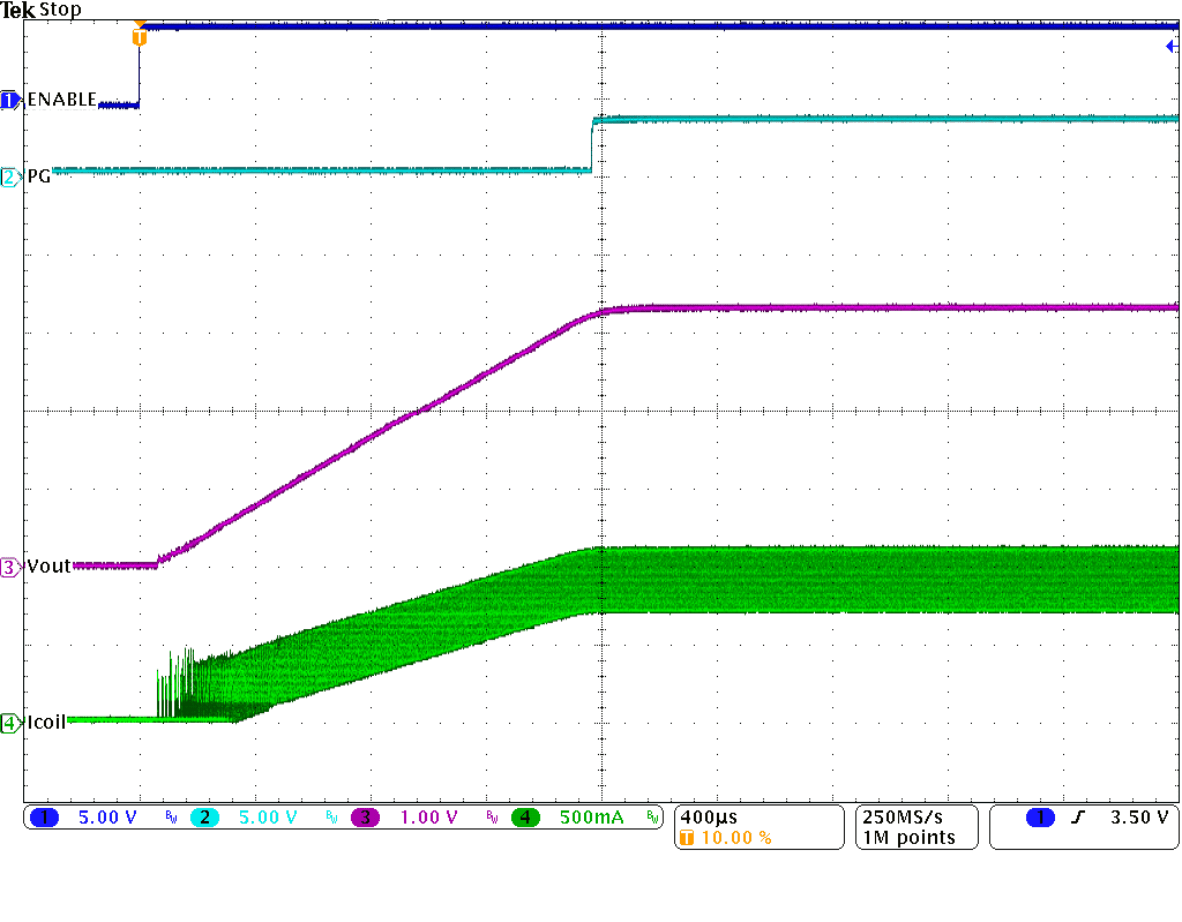Figure 33. Startup Into 1 A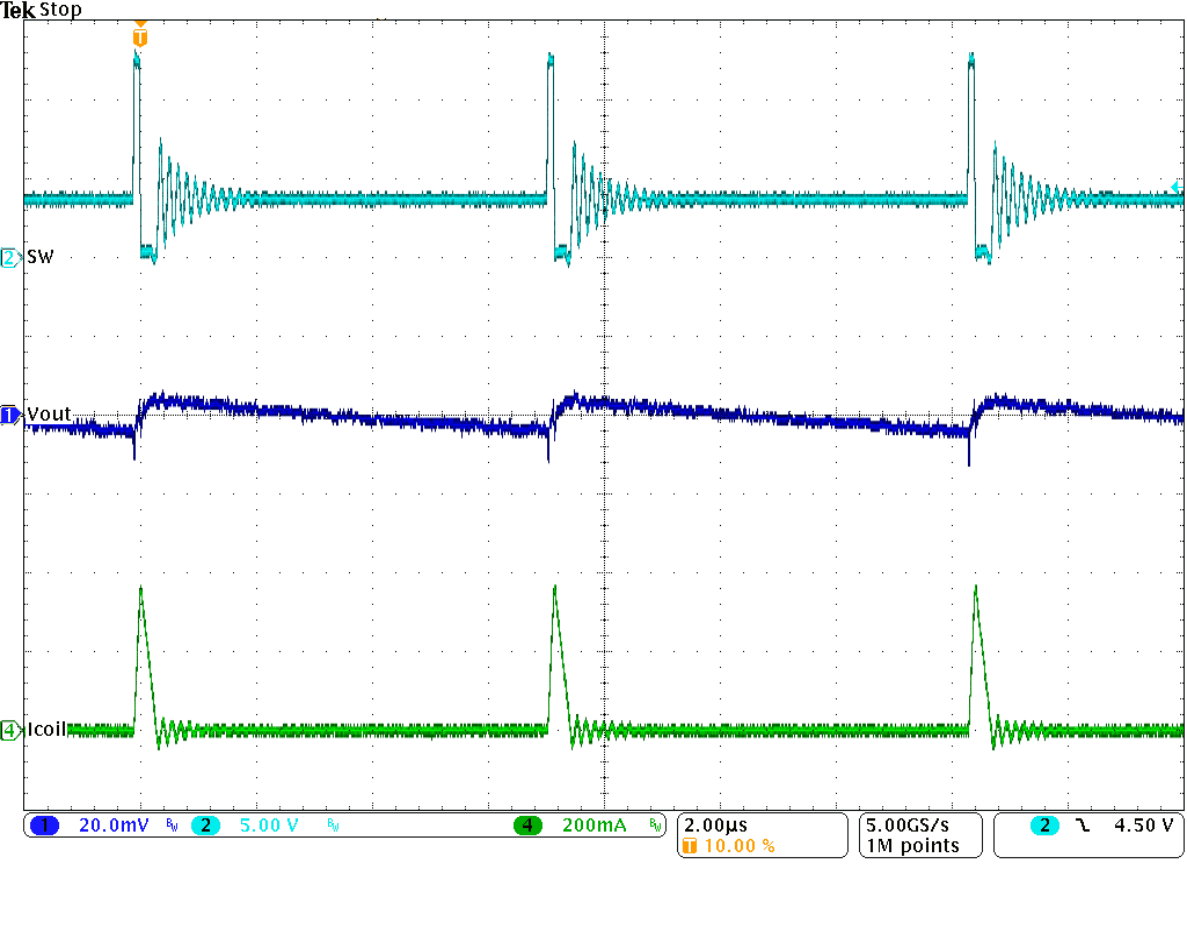Figure 35. Typical Operation in Power Save Mode
(IOUT = 10 mA)